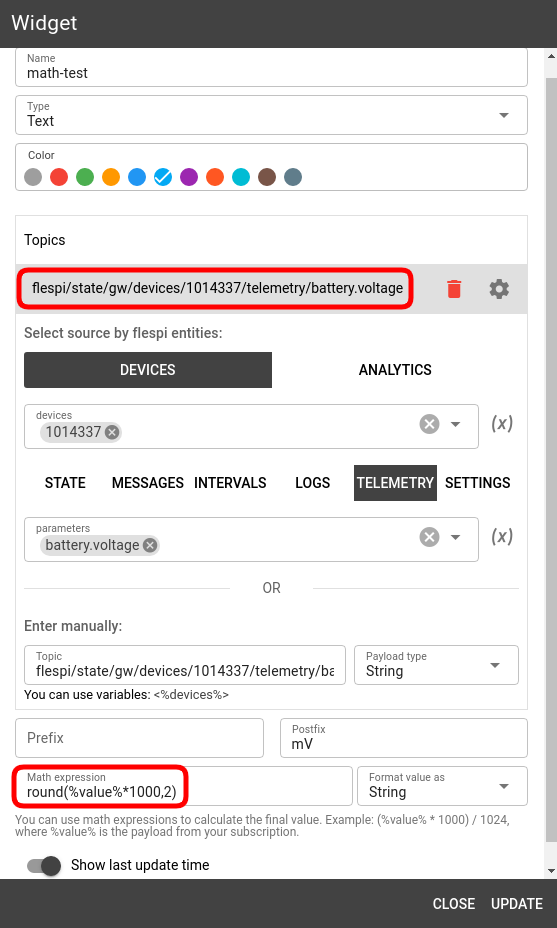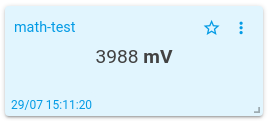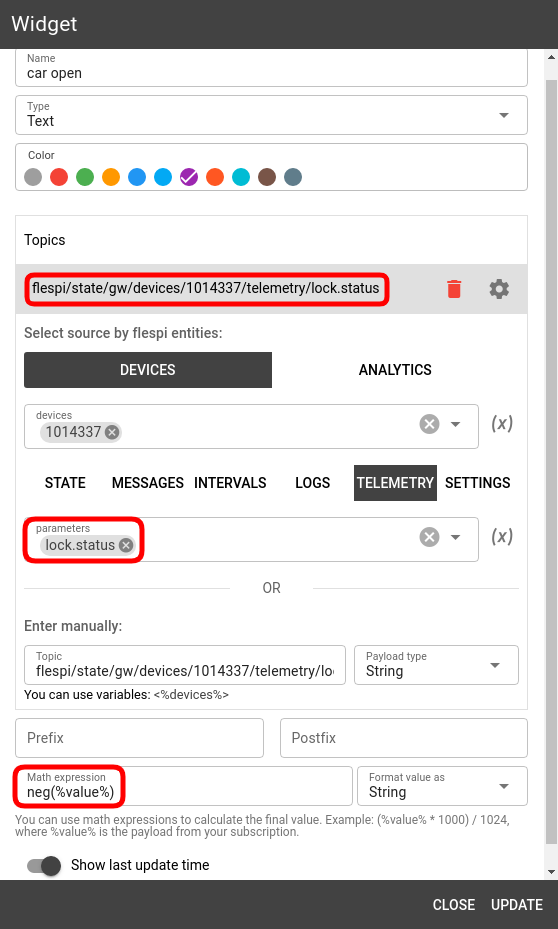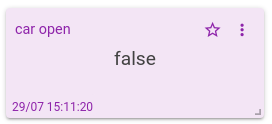# MQTT Tiles math capabilities

Some examples of the mathematical functionality supported in MQTT Tiles.

Most widgets in MQTT Tiles fully support the functionality of the math.js library that allows applying complex mathematical expressions to the parameter value extracted from the specified topic before showing it on the widget.

Here, we will showcase some of the possibilities that you can use in the Math expression field of all supporting widgets.

The basic arithmetic operations allow performing complex calculations on the original value.

Rounding functionality allows making the result more neat — use round(%value%, <number of decimal characters>) to arrive at the relevant accuracy.You can also do some operations on the boolean values. For instance, if we take the lock.status parameter but want to display the status on the “can open” widget, we need to invert the received value. To do so, we use the neg(%value%) function:## ***

The mathematical capabilities of MQTT Tiles are vast; most of them are the same or similar in syntax to the popular programming languages, so you shouldn't have problems using them.